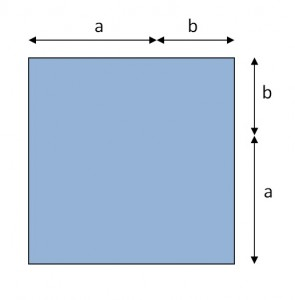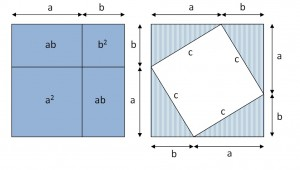# Picturing a Famous Theorem

In my last post, we saw how taking apart an area in different ways can lead to interesting results.  Let’s do that again.Here is the square with length (a + b):

(When we looked at binomial squares, I used x and y.  This time, I’m using a and b.  You’ll see why soon.)

Below, I have broken up the square two different ways.  On the left, you see the familiar shapes that we used to derive the formula for the square of a binomial.  And on the right, you see a square surrounded by four identical right triangles.This gives us two different ways to express the same area.  On the left, we have:

Area = (a + b)2 = a2 + 2ab + b2

On the right, we have one tilted square in the middle with side c, and 4 right triangles, each with a and b as their base and height.  We can add up their areas to find:

Area = (a + b)2 = c2 + 4 × ½ × a × b = c2 + 2ab

But those two areas are equal.  They are two different ways of chopping up the same square.  That means…

a2 + 2ab + b2 = c2 + 2ab

And if we subtract 2ab from both sides, we get a pretty well known result.

a2 + b2 = c2

where a and b are the legs and c is the hypotenuse  of a right triangle.

We use that theorem all the time.  Again, better with pictures.

All of these posts have been about using pictures to get an improved understanding of what the algebra is telling us.  These are ideas that I have been kicking around for a while so I thought I would share.  But they are leading up to something I’ll write about in the next post.  And I’ll reveal what got me started down this path again this year.

## One thought on “Picturing a Famous Theorem”

1. Congratulations on the new blog!

And the forthcoming book!